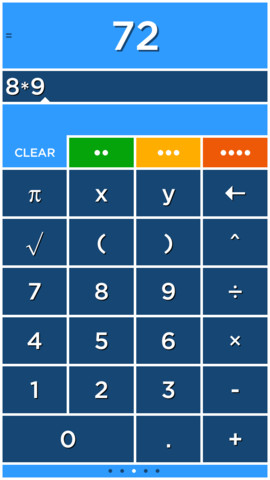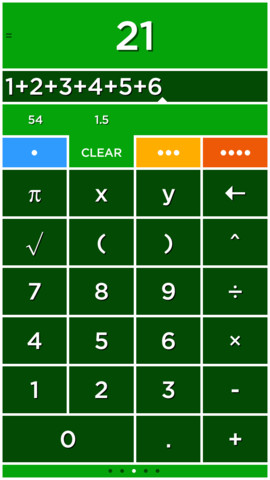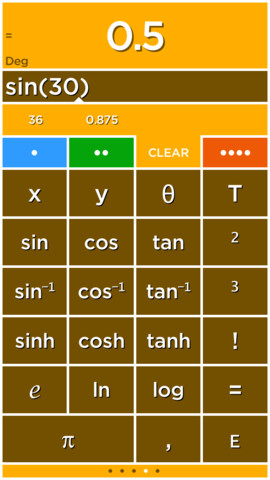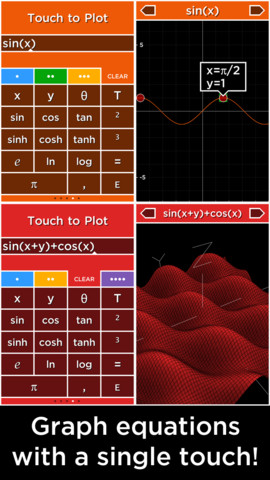5.0

# Solve - A colorful graphing calculator

• 小编评语
这款计算器在用起来的时候确实和常规计算器不太一样，用户可以在四种计算器中分别计算不同的算式，所有的结果都会暂时的存在计算器上方，用户可以通过直接点击来调用之前的存储结果。应用还支持自定义精确位数，支持科学计算器，解二元方程、三角函数方程。
• 内容摘要
Solve is a calculator like no other! Solve solves expressions as you type and features an innovative memory system to store and recall answers. Simply touch the answer to store it and touch the stored answer to use it in an expression.
Solve has four tabs that select four different calculators each with its own color. Multiply numbers, calculate a tip and find a square root all without clearing your results!
Plot graphs with a single touch! Solve plots 2D and 3D functions using the x, y and θ variables. When you use the x, y and θ variables in an equation the answer will say Touch to Plot. Touch this label to graph the equation.
GRAPHING
Plot functions using the x variable. For example, "y=sin(x)" is just entered as "sin(x)".
• cos(x)
• abs(x)
• x^2+2x+6
POLAR GRAPHING
Plot polar equations using the θ variable. For example, "r=2θ" is just entered as "2θ".
• 2θ
• sin(2θ)
3D GRAPHING
Plot 3D functions by using the x and y variables. For example, "z=x+y" is just entered as "x+y".
• cos(x)+sin(y)
• x^2+y^2
PLOT MULTIPLE GRAPHS
Graph multiple functions by separating each equation with a comma.
• sin(x),cos(x)
• x,x^2,x^3
TIME GRAPHING
The T variable is used to create animated or time plots.
• cos(x+T)
• sin(x+T)*x
• cos(x+T)+sin(y+T)

展开↓

• 版本中的新功能
Minor update.

展开↓

• 应用截图••#### 同类热门推荐

•••百‪度‬ 12.14.5 | 463.60MB
•FilzaJailed 3.1 | 13.20MB
•Panda Helper 2.2.3 | 14.60MB

#### 开发商的其他应用

•••MathStudio 7.3 | 5.70MB

#### 不可错过# 安装同步助手后能更便捷的下载此应用• 免费下载游戏和应用
• 快速安装APP
• 便捷管理、优化手机
A# 连接设备至同步助手，自动安装“Solve - A colorful graphing calculator”

### 安装步骤• 色情内容
• 赌博
• 暴力内容
• 反动内容
• 侵犯版权
• 木马病毒
• 恶意扣费
• 不良插件
• 无法安装或者启动
• 其他